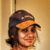# Skewness of values from 10 raster images

1422
4
01-26-2021 08:24 AMby
New Contributor III

Hi,

I am using ArcMap 10.8 version. I have 10 raster files with different data ranging from 0 to 1 for the same study area. I am interested to study how the data from 10 raster files are distributed in each pixel.

I want to calculate the skewness of the data in each pixels.

How can this be done in ArcMap?

Thank you!

3 Solutions

Accepted SolutionsbyMVP Esteemed Contributor

better solution,

get your multilayer raster out to a numpy array (RasterToNumPyArray), then use scipy's skew function

``````a = np.random.random_sample((10, 4, 3))  # 10 layer/band raster with 4 cols 3 rows
from scipy.stats import skew

sk_a = skew(a, axis=0)  # Calculate skewness over axis 0  ie local statistic
a0 = a[:, 0, 0]   # upper left corner over 10 layers
sk_a0 = skew(a0)  # check the skewness for the upper left over the 10 layers

sk_a
array([[-0.55202903,  0.9026473 , -0.22097936],
[ 0.05148204, -0.6512716 ,  0.16846484],
[ 0.93081464,  0.00802005,  0.04495503],
[-0.37445255,  0.18772049, -0.10078703]])

sk_a0  # confirm axis 0 calculation
-0.5520290295549968``````

... sort of retired...byMVP Esteemed Contributor

FYI

You can also bring the results back in.  In this example, I created a 10 "band" raster in NumPy and brought it back into ArcGIS ProAnd you can bring it back to numpy

``````b = arcpy.RasterToNumPyArray(pth)
b.shape
(10, 10, 10)

(a == b).all(-1) # ---- equality check, to make sure input and outputs are equal
array([[1, 1, 1, 1, 1, 1, 1, 1, 1, 1],
[1, 1, 1, 1, 1, 1, 1, 1, 1, 1],
[1, 1, 1, 1, 1, 1, 1, 1, 1, 1],
[1, 1, 1, 1, 1, 1, 1, 1, 1, 1],
[1, 1, 1, 1, 1, 1, 1, 1, 1, 1],
[1, 1, 1, 1, 1, 1, 1, 1, 1, 1],
[1, 1, 1, 1, 1, 1, 1, 1, 1, 1],
[1, 1, 1, 1, 1, 1, 1, 1, 1, 1],
[1, 1, 1, 1, 1, 1, 1, 1, 1, 1],
[1, 1, 1, 1, 1, 1, 1, 1, 1, 1]])``````

... sort of retired...byEsri Contributor

Hi Deepa,

If I understand your inputs and analysis objective correctly, you can do this easily using the following workflow. You don't need to create numpy arrays or use any other python package.

Please make sure to use the correct formula for calculating skewness in the map algebra expression below.

Thanks

Noman

#### Calculating Pearson's second skewness coefficient (median skewness)

Input: 10 single band rasters or 1 multiband raster with 10 bands

Steps (using Spatial Analyst tools and operators)

1. Run CellStatistics 3 times to create mean, median, and standard deviation outputs as cs_mean, cs_median, cs_stddev respectively.
2. Execute the following map algebra expression in Raster Calculator or in Python using arcpy.sa molule.

out_skewness = 3 * (cs_meancs_median) / cs_stddev

4 RepliesbyMVP Esteemed Contributor

Local statistics Cell Statistics (Spatial Analyst)—ArcGIS Pro | Documentation

can easily give you mean, median and standard deviation.

How difficult the next step would be would depend on which measure of skewness you wanted

Skewness - Wikipedia

Pearson's second skewness coefficient might suffice

... sort of retired...byMVP Esteemed Contributor

better solution,

get your multilayer raster out to a numpy array (RasterToNumPyArray), then use scipy's skew function

``````a = np.random.random_sample((10, 4, 3))  # 10 layer/band raster with 4 cols 3 rows
from scipy.stats import skew

sk_a = skew(a, axis=0)  # Calculate skewness over axis 0  ie local statistic
a0 = a[:, 0, 0]   # upper left corner over 10 layers
sk_a0 = skew(a0)  # check the skewness for the upper left over the 10 layers

sk_a
array([[-0.55202903,  0.9026473 , -0.22097936],
[ 0.05148204, -0.6512716 ,  0.16846484],
[ 0.93081464,  0.00802005,  0.04495503],
[-0.37445255,  0.18772049, -0.10078703]])

sk_a0  # confirm axis 0 calculation
-0.5520290295549968``````

... sort of retired...byMVP Esteemed Contributor

FYI

You can also bring the results back in.  In this example, I created a 10 "band" raster in NumPy and brought it back into ArcGIS ProAnd you can bring it back to numpy

``````b = arcpy.RasterToNumPyArray(pth)
b.shape
(10, 10, 10)

(a == b).all(-1) # ---- equality check, to make sure input and outputs are equal
array([[1, 1, 1, 1, 1, 1, 1, 1, 1, 1],
[1, 1, 1, 1, 1, 1, 1, 1, 1, 1],
[1, 1, 1, 1, 1, 1, 1, 1, 1, 1],
[1, 1, 1, 1, 1, 1, 1, 1, 1, 1],
[1, 1, 1, 1, 1, 1, 1, 1, 1, 1],
[1, 1, 1, 1, 1, 1, 1, 1, 1, 1],
[1, 1, 1, 1, 1, 1, 1, 1, 1, 1],
[1, 1, 1, 1, 1, 1, 1, 1, 1, 1],
[1, 1, 1, 1, 1, 1, 1, 1, 1, 1],
[1, 1, 1, 1, 1, 1, 1, 1, 1, 1]])``````

... sort of retired...byEsri Contributor

Hi Deepa,

If I understand your inputs and analysis objective correctly, you can do this easily using the following workflow. You don't need to create numpy arrays or use any other python package.

Please make sure to use the correct formula for calculating skewness in the map algebra expression below.

Thanks

Noman

#### Calculating Pearson's second skewness coefficient (median skewness)

Input: 10 single band rasters or 1 multiband raster with 10 bands

Steps (using Spatial Analyst tools and operators)

1. Run CellStatistics 3 times to create mean, median, and standard deviation outputs as cs_mean, cs_median, cs_stddev respectively.
2. Execute the following map algebra expression in Raster Calculator or in Python using arcpy.sa molule.

out_skewness = 3 * (cs_meancs_median) / cs_stddev